Open in App
Not now

• Difficulty Level : Medium
• Last Updated : 03 Dec, 2021

Prerequisite: Designing Finite Automata

DFA (Deterministic Finite Automata or Acceptor) is a finite state machine that accepts or rejects strings of symbols. DFA accepts the string if it reaches the final state otherwise it rejects it. In these types of problems, we have some given parameters according to which we should design DFA.

Problem– Construct a DFA that either starts with aa or with bb from input (a,b).

Solution– In this problem, two parameters are given:

or

This means, the resulting DFA should accept the strings such as aa, bb, aab, bba, aaa, bbb…. etc but it should not accept strings such as a, b, ba, bab, abb, abbaba… etc.

### Designing DFA Step-by-Step:

Step-1: Take initial state qo, and smallest possible string are aa and bb if qo takes ‘a’ as the first input alphabet it goes to state q1 and if qo takes ‘b’ as the first input alphabet it goes to state q3.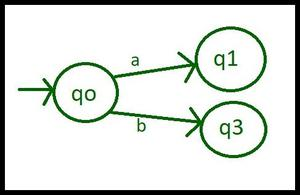Step 1 for DFA construction

Step-2: Now think about state q1, if it takes input alphabet ‘b’, it breaks our condition of  ‘aa’ but if takes input alphabet ‘a’ it makes an acceptable string, and now it goes to state q2 which is set to the final state.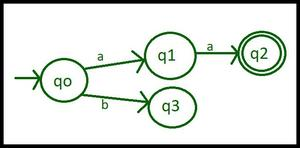Step 2 for DFA construction

Step-3: On state q3 if it takes input alphabet ‘a’, it breaks our condition of  ‘bb’ but if takes input alphabet ‘b’ it makes an acceptable string, and now it goes to state q2 which is set to the final state.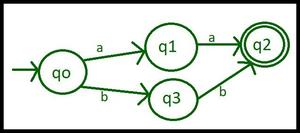Step 3 for DFA construction

Step-4: If input alphabet ‘a’ of state q3 breaks the condition and Input alphabet ‘b’ of state q1 breaks the condition so they go to some dead state(D).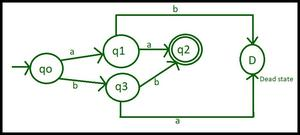Step 4 for DFA construction

Step-5: Till now, our machine accepts strings that starts from ‘aa’ or from ‘bb’ but we also have to take care of all the symbols after the string has begun from ‘aa’ or ‘bb’ and hence we introduce ourselves loops in q2(final state) and D(dead state).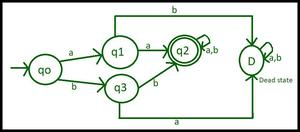Step 5 for DFA construction

### Transition table and transition rules for the above diagram:

The Finite set of states = {qo, q1, q2, q3, D}

In the transition table, the initial state(qo) is represented byand the final state is q2 and has two circles.

Set of input alphabets = {a, b}

### Transition Table

My Personal Notes arrow_drop_up